[BACK]
 Computer Systems Science & EngineeringDOI:10.32604/csse.2022.018962Article

Obtaining Crisp Priorities for Triangular and Trapezoidal Fuzzy Judgments

1Computer Science and Engineering Department, Thapar Institute of Engineering and Technology, Patiala, Punjab, India
2Computer Science and Engineering, University Institute of Engineering and Technology, Panjab University, Chandigarh, India
*Corresponding Author: Anshu Parashar. Email: aparashar@thapar.edu
Received: 27 March 2021; Accepted: 03 May 2021

Abstract: This paper proposes anoptimal fuzzy-based model for obtaining crisp priorities for Fuzzy-AHP comparison matrices. Crisp judgments cannot be given for real-life situations, as most of these include some level of fuzziness and complexity. In these situations, judgments are represented by the set of fuzzy numbers. Most of the fuzzy optimization models derive crisp priorities for judgments represented with Triangular Fuzzy Numbers (TFNs) only. They do not work for other types of Triangular Shaped Fuzzy Numbers (TSFNs) and Trapezoidal Fuzzy Numbers (TrFNs). To overcome this problem, a sum of squared error (SSE) based optimization model is proposed. Unlike some other methods, the proposed model derives crisp weights from all of the above-mentioned fuzzy judgments. A fuzzy number is simulated using the Monte Carlo method. A threshold-based constraint is also applied to minimize the deviation from the initial judgments. Genetic Algorithm (GA) is used to solve the optimization model. We have also conducted casestudiesto show the proposed approach’s advantages over the existingmethods. Results show that the proposed model outperforms other models to minimize SSE and deviation from initial judgments. Thus, the proposed model can be applied in various real time scenarios as it can reduce the SSE value upto 29% compared to the existing studies.

Keywords: Analytic hierarchy process; comparison matrices; priority vectors; fuzzy judgments; triangular fuzzy numbers; triangular-shaped fuzzy numbers; trapezoidal fuzzy numbers

1  Introduction

Multi-Criteria decision-making (MCDM) methods are used to solve complex decision-making systems. Rao  has listed many decision-making methods like AHP  ELECTRE , TOPSIS , PROMETHEE  and GRA  etc. AHP is the most popular method among these MCDM methods. Triantaphyllou and Lin  discussed advantages of AHP in many real-life selection problems. AHP had been integrated with other theories and practices by researchers to solve many decision-making problems in MCDM scenarios . Zhang et al.  proposed a method for prioritization using additive and multiplicative relative deviation interconnection in pairwise comparison matrices. Aguarona et al.  proposed a novel method for improving the inconsistency of the GCI of AHP by a reevaluation of judgments. Olabanji et al.  proposed MADM method to choose the best design for the Reconfigurable Assembly Fixture (RAF) from the set of choices. They considered the better of two techniques Fuzzy AHP and Fuzzy Weighted Average for choosing among various designs. Gundogdu et al.  proposed a fuzzy spherical AHP method to select renewable energy sites. Guler et al.  proposed a novel method based on Fuzzy AHP and Geographic Information System to select stations for electric vehicle charging. Sarkar et al.  presented a hybrid technique consisting of Fuzzy AHP and TOPSIS to solve transport management’s decision-making issues. They have used Pythagorean fuzzy sets to overcome the limitation of existing distance measuring techniques. Crisp judgments cannot be given in many real-life scenarios as most of these include some level of fuzziness and complexity. In such situations, judgments are given in fuzzy numbers . Derivation of crisp weights from the fuzzy numbers is a difficult task . Triangular fuzzy weights have been obtained from the fuzzy triangular matrix by the logarithmic least squares method (LLSM) . Buckley  derived fuzzy weights from trapezoidal fuzzy numbers. Boender et al.  proposed a technique on normalizing the local priorities and showed that this normalizing technique in LLSM might result in irrational weights. Generalized pseudo-inverse method has been applied by Kwiesielewicz  to obtain a consistent and geometrically normalized solution. All of the models mentioned above derive fuzzy weights. Therefore, they also require additional aggregation and ranking processes. Extent analysis method  removes these requirements and derives crisp priorities for the fuzzy comparison matrices. Wang et al.  have found that this method does not estimate true weights and assigns irrational zero weights to useful attributes in some scenarios. Interval priorities weights are derived from fuzzy comparison matrices by some methods . But these methods also require additional fuzzy ranking procedures.

Mikhailov  has derived crisp weights using a linear fuzzy prioritization programming method using a series of interval judgments. This method removes the requirement of the ranking process but still requires the aggregation process. Therefore, Mikhailov  also proposed a non-linear fuzzy prioritization (fuzzy optimization) method to remove the aggregation process. Wang et al.  proposed a new optimization model for fuzzy judgments. The objective function’s degree was varied from 2 to 10. Javanbarget al.  have solved three examples with the same objective function of degree . Goyal et al.  proposed a constraint for this objective function to improve its consistency. New methods presented by Goyal et al. in  and Goyal et al.  also derive more consistent weights than the fuzzy optimization model shown in Javanbarg et al. .

All these fuzzy optimization models derive crisp weights for Triangular Fuzzy Numbers (TFNs) only in the literature presented above. Mohtashami  proposed a Modified Fuzzy Logarithmic Least Square Model (MFLLSM) to derive priorities from TFNs, Triangular Shaped Fuzzy Numbers (TSFNs), and Trapezoidal Fuzzy Numbers (TrFNs). Evaluation of trapezoidal fuzzy numbers on AHP based solution of multi-objective programming problems for group decision making is given by AkbasandDalkilic .

After introducing some preliminary concepts for the fuzzy judgments in comparison pairwise matrices in Section 2, the following contributions are claimed:

• A novel Sum of Squared Error (SSE) based fuzzy optimization model is proposed. The simulation of the fuzzy number using Monte Carlo’s method and the threshold-based constraint is also presented.

• Further empirical analysis has been conducted based on eight case studiesto compare the proposed SSE-based model with existing models. It has been proved that the proposed model performs better than the existing Mohtashami’s model .

To the best of the author’s knowledge, no other fuzzy optimization model has been proposed till now to derive crisp weights for these types of fuzzy judgments that can surpass the work done by Mohtashami .

2  Materials and Methods

In this section, we have discussed how the various fuzzy judgments can be represented.

2.1 Fuzzy Judgments

For modeling many real-time applications, a decision-maker cannot always give crisp judgments. In these situations, judgments are illustrated by fuzzy numbers . Fuzzy judgments can be of the following forms:

Triangular Fuzzy Number (TFN): A TFN is represented as (l,m,u) where l, m, and u denote the lowest value, the most promising value uppermost possible value, respectively. Membership function of a TFN is represented in Eq. (1) .

μN~(x)={xlml,lxm1pcuxum,mxu1pc0,otherwise (1)

Triangular Shaped Fuzzy Numbers (TSFNs): These fuzzy numbers are represented similarly to TFN except the member function does not increase or decrease linearly. A TSFN is defined as shown in Eq. (2). If the values of δ and χ are equal to one, then this membership function becomes the function of TFN. Fig. 1. 1 depicts the asymmetric membership function for TSFN with δ=3 and χ=5. From Fig. 1, it can be visible that there is more intent of the decision-maker towards l rather than u. If the values of χ and δ are equal, then the shape of the membership function is symmetric with the decision-maker’s equal intent towards both l and u.

μN~(x)={(xlml)δ,lxm1pc(uxum)χ,mxu1pc0,otherwise (2)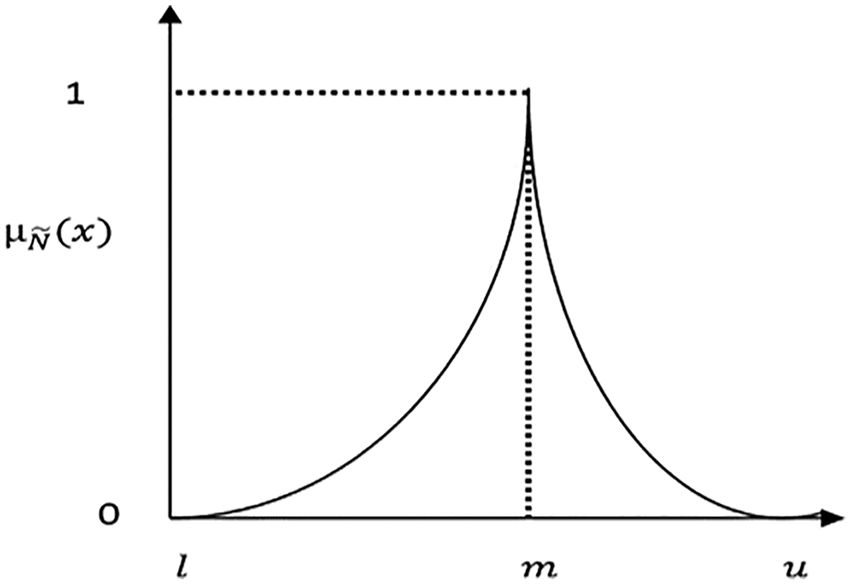Figure 1: Triangular shaped Fuzzy Number with δ<χ

Trapezoidal Fuzzy Number: In this type of membership function, there are two midpoints between the lower and upper limits and are represented as (l, m1, m2, u). The membership function for the trapezoidal fuzzy numbers (TrFNs) is shown in Eq. (3). Fig. 2. shows the graphical representation of TrFN.

μN~(x)={xlm1l,lxm11pcuxum2,m2xu1pc1,m1xm21pc0,otherwise (3)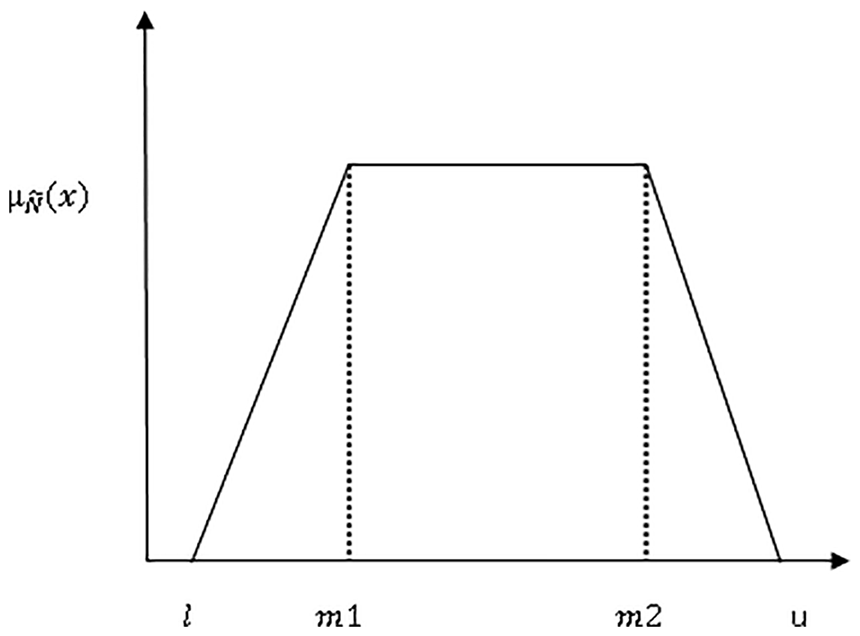Figure 2: Trapezoidal Fuzzy Number

Trapezoidal Shaped Fuzzy Number (TrSFN): This type of fuzzy number is similar to TrFN except that the membership function does not increase or decrease linearly. The TrSFN membership function is shown in Eq. (4). If the values of δ and χ are equal to one, then this membership function becomes the function of TrFN.

μN~(x)={(xlm1l)δ,lxm11pc(uxum2)χ,m2xu1pc1,m1xm21pc0,otherwise (4)

The main challenge is to devise a model that can derive crisp priorities from all these types of fuzzy judgments. In the next section, the proposed fuzzy optimization model for obtaining crisp priorities is presented.

2.2 Proposed Work

This section presents a novel fuzzy prioritization method for deriving crisp priorities from fuzzy pairwise comparison matrices. Here, the proposed SSE-based fuzzy optimization model is discussed and the constraint applied to the fuzzy optimization model for minimum deviation from initial judgmentsis also presented. Further, the fuzzy number simulation method is elaborated, and the overall working of the proposed work has been discussed.

2.2.1 Fuzzy Optimization Model

In this paper, a new Sum of Squared Error (SSE) based fuzzy optimization model is presented as a nonlinear system. Crisp weights (w1, w2, …, wn) of n criteria are derived from fuzzy judgments (a~ij ) in the comparison matrices as follows:

min J(w1,w2,,wn)=mini=1nj=1n(a~ij(wiwj))2(ij) (5)

subject to k=1nwk=1,k=1,2,n. where wi and wj> 0

The weight ratio (wi/wj) must satisfy the following inequality:

lij~wi/wj~uij (6)

lij and uij represent the lower and upper limits of fuzzy judgments. The symbol ~ represents the fuzzy inequalities. For a consistent comparison matrix, solutions satisfying lij  ≤ wi/wj ≤ uij can be found. But for inconsistent matrices, there will be no solution to satisfying this inequality simultaneously. Therefore, the fuzzy inequality lij~wi/wj~uij is applied to find the crisp priorities that satisfy the inequality as well as it can. To find the weight ratios wi/wj that deviate minimum for this inequality, a constraint is proposed. a~ij represents the fuzzy judgments in the form of TFNs, TSFNs, TrFNs, and TrSFNs. In the case of TFN and TrFN, the value of a~ij assumed as the center of the fuzzy number. In other cases, the value of a~ij represents the objective function obtained by Monte Carlo simulation.

2.2.2 Monte Carlo’s Method for Fuzzy Number Simulating

The value of a~ij is obtained, as shown in Algorithm 1. This algorithm is a modified version of the Monte Carlo method for fuzzy number simulation presented by Mohtashami . Monte Carlo simulation is used to generate the fuzzy number based on algorithm 1.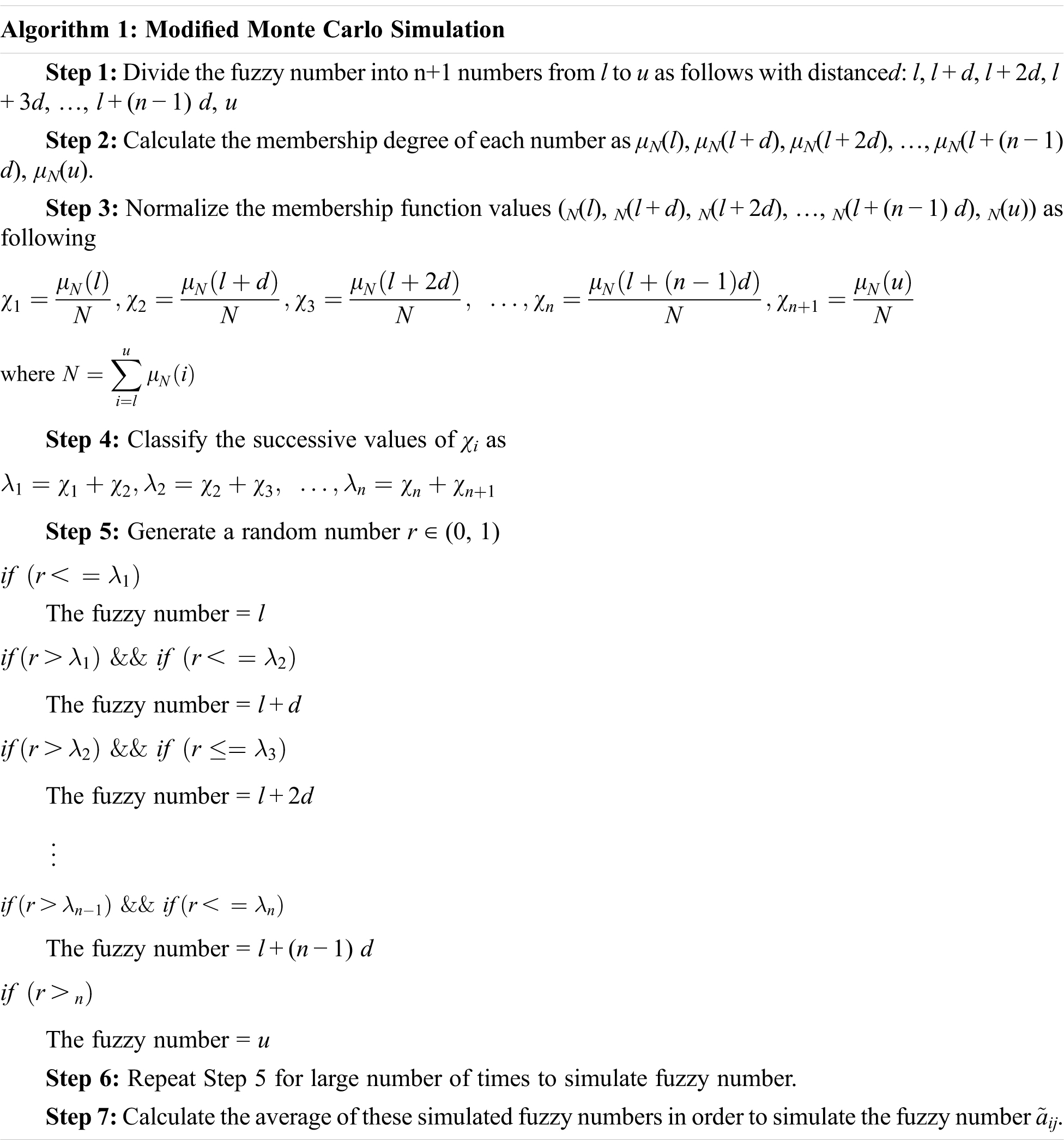To check the effectiveness of this proposed Model, graphs for different types of fuzzy numbers have been drawn considering the frequency (count) of the simulated fuzzy number. If the values of δ and χ are equal to one, then it is a TFN otherwise, the number is TSFN. The values of (l,m,u) are taken as (1,2,3). Similarly, this method can be extended to Trapezoidal Fuzzy Numbers (TrFN). Fig. 3. shows the shape obtained for a TrFN with values (1, 1.5, 2.5, 3), which is similar to the actual shape.

lijwi/wjuij (7)

The point to be noted here is that this inequality is not ~ as mentioned in Eq. (6). But, for inconsistent pairwise matrices, no set of priority vectors can simultaneously satisfy this inequality. Therefore, fuzzy inequality is used in Eq. (6) to allow some deviation. To achieve minimum violation from the Eq. (7), the constraint shown in Eq. (8) is applied.Figure 3: Simulation of trapezoidal number based on algorithm 1

2.2.3 Violation from Initial Judgments

Each priority vector (crisp weight) should satisfy the constraint defined in Eq. (6). The proposed algorithm first tries to obtain the crisp weights by satisfying the following inequality

lijαwiwjuij+α (8)

α represents the threshold value of the allowed deviation for the optimization problem. If the solution is feasible, then the crisp weights are derived. Otherwise, the optimization process is repeated and α is increased with a small value of 0.1. After obtaining the feasible crisp weights ( w1 , w2,,wn) deviation from initial judgments (ε) can be calculated as follows:

ϵ=max(wiwjlij,uijwiwj) where i,j=1,2,3,n. (9)

Thus, the crisp weights deviate with ε from the initial judgments far less than the existing models shown in Section 4.

2.2.4 Working of the Proposed Model

The working of the proposed model is shown in Fig. 4. Firstly, the fuzzy number is simulated, as mentioned in above mentioned algorithm.Figure 4: Working of the proposed technique

Then, the crisp weights are derived using the fuzzy optimization model, as shown in Eq. (5). To check the deviation from initial weights, a threshold-based constraint shown in Eq. (8) is applied. The value of α is set to zero initially and is increased by 0.1 until feasible weights are obtained. Genetic Algorithm (GA) [45,46] is used to solve this optimization problem with both population size and number of iterations equal to 300 and 350 respectively. Rank scaling is used as a fitness scaling function, and Uniform Stochastic Sampling is used to select parents. The heuristic operator is applied for crossover operation while adaptive feasible mutation is applied for the mutation process. We have solved this objective function by GA method with significantly large population size of 300 and 350 iterations for each example in Matlab. Any optimization algorithm can be used to solve this optimization problem. The time complexity is increased in case of finding crisp weights only. Obtaining the crisp weights is a one-time process only. So, it will have no impact on the selection process. So, the proposed model can be easily applied in real world problems.

3  Case Studies and Result Analysis

To evaluate the proposed model, eight case studies are solved. As discussed earlier, among the fuzzy optimization models, only Mohtashami’s model  derive crisp weights for all the TFNs, TSFNs and TrFNs.

Case-1:

In this example, a perfectly consistent comparison pairwise matrix with only two elements is considered. In comparison to the second element, the first element is more important and is represented by a TFN a~12 = (1,2,3). Crisp weights obtained by different methods are shown in Tab. 1. Results show that the difference between crisp weights obtained by the proposed fuzzy optimization model and the existing models is inconsiderable. Therefore, results obtained by the proposed model are acceptable for this consistent matrix.Case-2:

Consider the comparison matrix of three elements represented by TFNs as a~13 = (4, 5, 6), a~21 = (2, 3, 4) and a~23 = (6, 7, 8). For this, the value of SSE is more as compared to Mohtashami’s  model as shown in Tab. 2. But the value of ε is not zero with Motashami’s model. The value of SSE is more with the proposed model as it minimizes the SSE by constraining the deviation (ε) from the judgments. Therefore, the value of ε is zero with the proposed model. The proposed derives similar priority vectors (crisp weights) as of Non-Linear FPP model. Linear FPP performs poorly in terms of SSE and ε. For illustration purpose, the weights are also derived with α (threshold value) = 0.1. The results of the proposed model are better than Mohtashami’s model. Therefore, the proposed model outperforms all the other models for this example.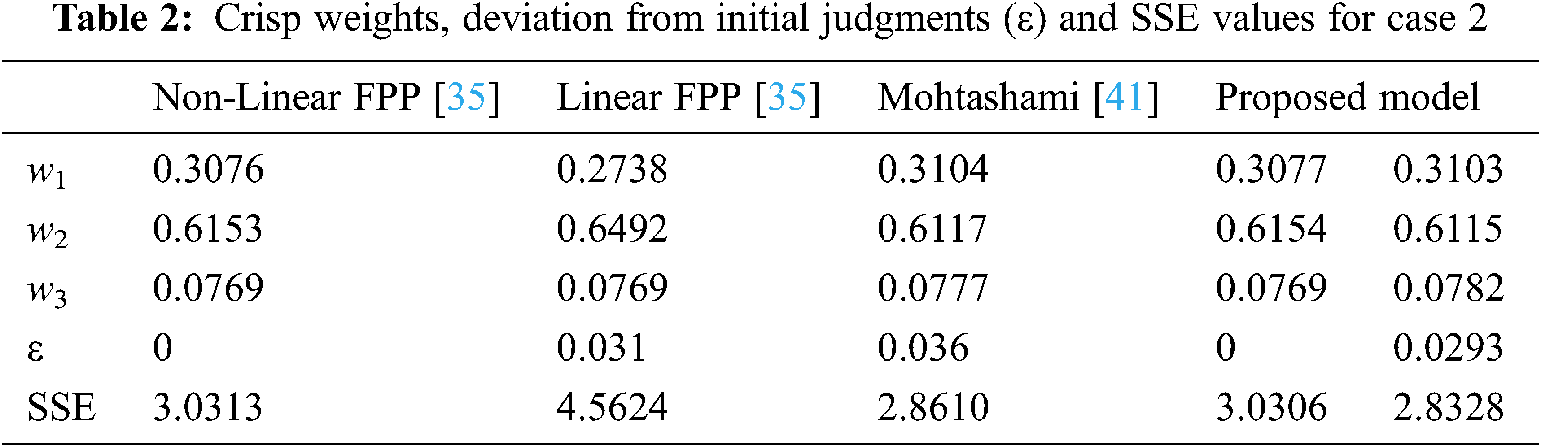Case-3:

In this example, judgments of the comparison matrices of four elements are given as a~12 = (7, 8, 9), a~13 = (3, 4, 5), a~14 = (6, 7, 8), as a~32 = (4, 5, 6), a~34 = (7, 8, 9) and a~42 = (3, 4, 5). Tab. 3. depicts the values of SSE and ε. Results show the superiority of the proposed model over all the other models. The value of SSE in Mohtashami model is more than the Linear FPP model. But with the proposed model, lesser values of SSE and ε are obtained as compared to all the considered models.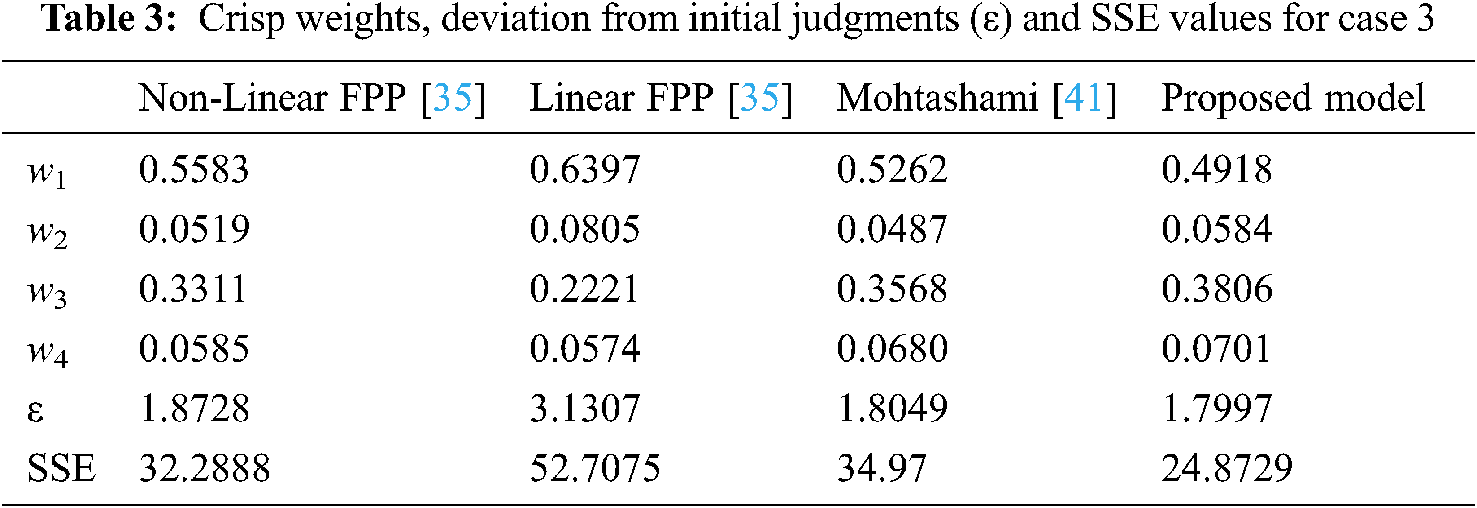In all the above examples, triangular fuzzy numbers are used. But, the proposed optimization model can work for trapezoidal numbers as well. The followingcases demonstrate the usefulness of the proposed model by taking the judgments in the comparison matrices in the form of TrFNs. As discussed earlier, only Mohtashami’s model  can derive crisp weights from the TrFN and TSFN judgments out of all the fuzzy optimisation models. Therefore, only Linear FPP and Mohtashami’s models are considered compared to the proposed fuzzy optimization model.

Case-4:

Consider the comparison matrix of four elements where the judgments are represented in the form of TrFNs as a~12 = (4, 9/2, 11/2, 6), a~14 = (7, 15/2, 17/2, 9), a~31 = (3, 7/2, 9/2, 5), a~32 = (7, 15/2, 17/2, 9), a~34 = (6, 13/2, 15/2, 8) and a~42 = (3, 7/2, 9/2, 5). Results show that the proposed model derives crisp weights with lesser values of SSE and ε as compared to Linear FPP and Mohtashami’s model as shown in Tab. 4.Case-5:

To demonstrate the superiority of the proposed fuzzy optimization model over the other models, another example where judgments are represented in the form of TrFNs is considered. Suppose the judgments of the comparison matrix are as a~12 = (2, 5/2, 7/2, 4), a~13 = (5, 11/2, 13/2, 7), a~14 = (6, 13/2, 15/2, 8), a~32 = (6, 13/2, 15/2, 8), a~34 = (7, 15/2, 17/2, 9) and a~42 = (3, 7/2, 9/2, 5). Tab. 5. depicts the values of crisp weights, SSE and ε. Tab. 5 depicts the values of crisp weights, SSE and ε. The proposed model shows its superiority to the other models in terms of ε and SSE values. Therefore, these two examples demonstrate that the proposed model performs better than the other models for judgments represented in the form of TrFNs.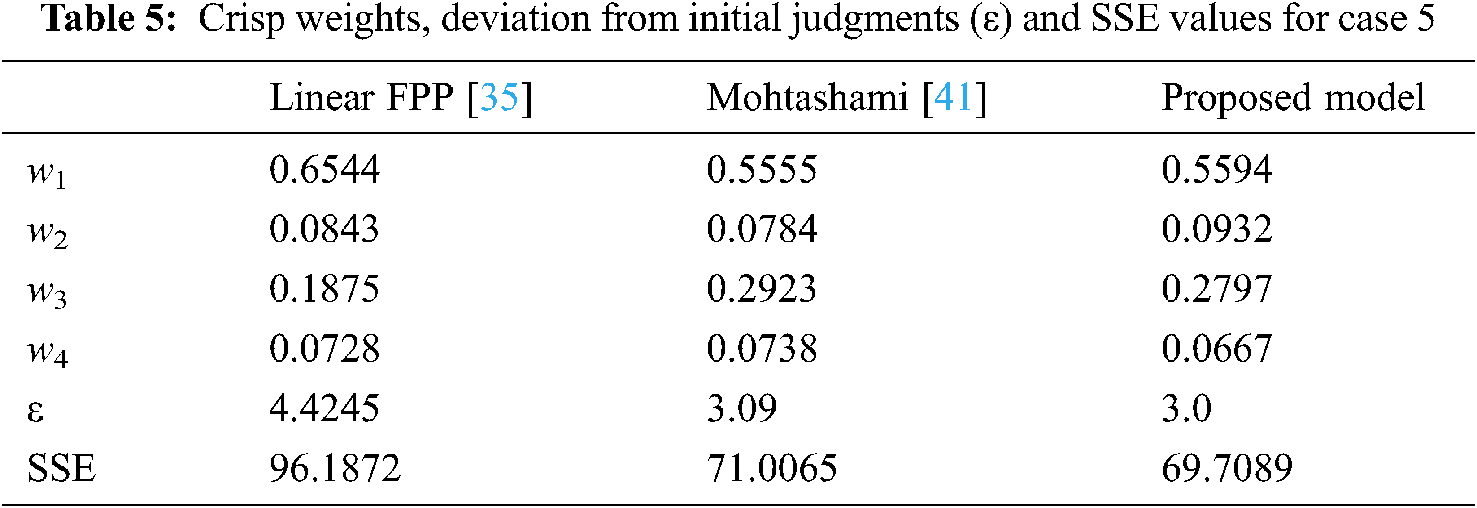Till now the fuzzy judgments considered in this manuscript are symmetric like TFNs and TrFNs. But in some situations, a decision-maker makes the judgments of asymmetric nature like TSFNs and TrSFNs. If the values of δ and χ are equal to one, then it demonstrates TFN otherwise it will act as TSFN.

Case-6:

Consider a comparison matrix of four elements in which judgments are represented by a~12 = (3, 4, 5), a~14 = (7, 8, 9) δ=3,χ=3 as TSFN; a~31 = (4, 5, 6) as TFN; a~32 = (7, 8, 9) δ=3,χ=1/3 as TSFN, a~34 = (3, 4, 5) as TFN and a~42 = (1, 2, 3) δ = 1/3, χ = 3 as TSFN. Tab. 6 depicts the values of crisp weights, SSE and ε. The value of SSE with the proposed model is 36.4661 as compared to 37.5998 in Mohtashami’s model. Values of SSE and ε in Linear FPP are very high. Results show that the proposed model outperforms all these models as depicted in Tab. 6.Case-7:

To show the proposed model’s effectiveness, another example of comparison matrix with four elements is considered. Judgments are represented as by a~12 = (3, 4, 5) δ=1/3,χ=3 as TSFN, a~31 = (5, 6, 7) as TFN; a~32 = (7, 8, 9) δ=3,χ=1/3 as TSFN; a~34 = (3, 4, 5) δ=3,χ=3 as TSFN, a~41 = (7, 8, 9) as TFN and a~42 = (4, 5, 6) δ=1/3,χ=3 as TSFN. Linear FPP model performs poorly as shown in Tab. 7. The values of SSE and ε with the Mohtashami’s model are 39.4676 and 1.9846, respectively. These values are comparatively more than the proposed model’s value of 37.4647 and 1.6, respectively. Thus, the proposed model outperforms all the other considered models.Case-8:

In the last example, consider the comparison matrix of four elements as a~13 = (3, 4, 5) δ=1/3,χ=3 as TSFN, a~14 = (3, 4, 5) δ=1/3,χ=3 as TSFN; a~21 = (5, 6, 7) δ=3,χ=3 as TSFN; a~23 = (1, 2, 3) δ=1/3,χ=3 as TSFN, a~24 = (3, 4, 5) as TFN and a~43 = (7, 8, 9) δ=1/3,χ=3 as TSFN. Tab. 8. depicts the values of SSE and ε of different models for deriving crisp weights. In this case, the SSE value for the proposed model is more as compared to Mohtashami’s model. But the value of ε is lesser with the proposed model. Similarly, when the value of threshold limit (α) is increased, the values of SSE and ε are lesser with the proposed model is compared to Mohtashami’s model. Thus the proposed model is superior to the other models for this example also. In all the eightexamples the proposed model performs better than the other models. Therefore, crisp weights can be derived from different types of fuzzy judgments. This model can be applied in various real-life applications like operation research, material selection, and handover in wireless networks, cloud computing, and other managerial and engineering problems.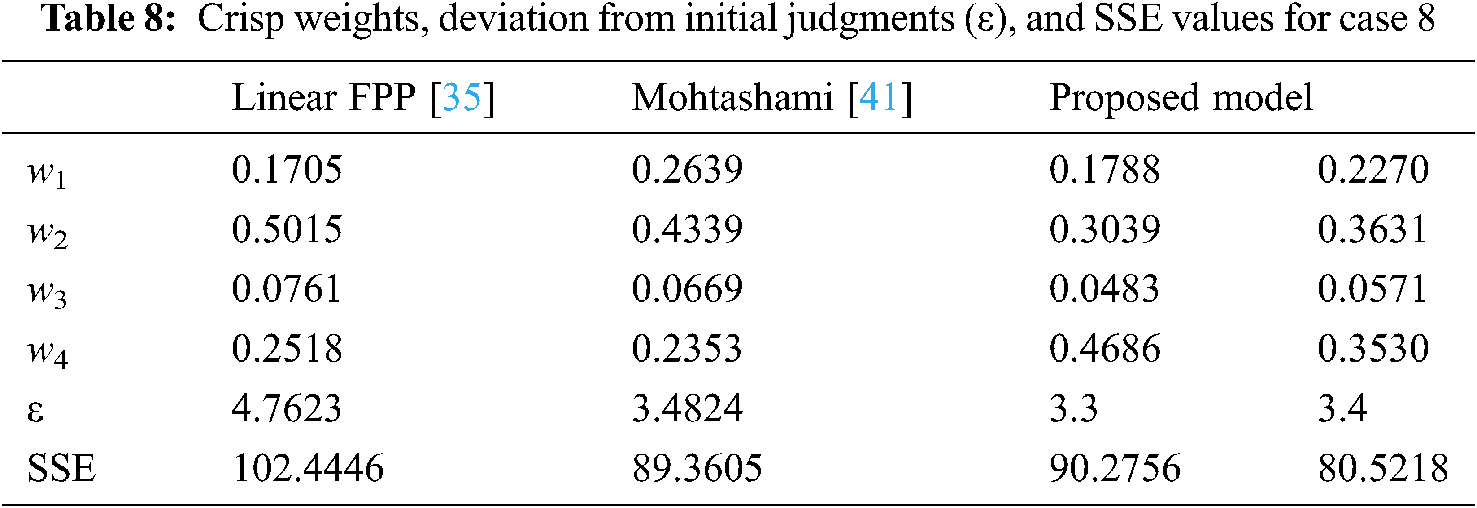4  Conclusion

This paper presents a novel Sum of Squared Error (SSE) based fuzzy optimization model to derive crisp weights for fuzzy pairwise comparison matrices. The proposed optimization model can derive priorities for Triangular Fuzzy Numbers (TFNs), Trapezoidal Fuzzy Numbers (TrFNs), Triangular Shaped Fuzzy (TSFNs), and Trapezoidal Shaped Fuzzy Numbers (TSFNs) unlike most of the optimization models that derive weights in the case of TFNs only. Simulation of the fuzzy number is done by Monte Carlo’s method. The proposed model minimizes the SSE value and deviation from judgments ischecked by applying a threshold-based constraint.Eightexamples are illustrated that include examples of consistent and inconsistent fuzzy judgments represented by TFNs, TrFNs and TSFNs. Results show that the proposed model outperforms other models for all theabove mentioned cases.

Analytic Hierarchy Process (AHP) can be used in many decision-making problems in various fields like healthcare  operation research , management , engineering , etc. Therefore, the proposed model has utility in all these decision-making systems where decision makers cannot give crisp weights. The proposed model’s main limitation is that it considers the judgments as type 1 fuzzy sets only. This work can be further extended to obtain crisp priorities from type 2 fuzzy sets. Also methods to measure theconsistency for triangular shaped and trapezoidal fuzzy comparison matrices can be measured.

Funding Statement: The authors received no specific funding for this study.

Conflicts of Interest: The authors declare that they have no conflicts of interest to report regarding the present study.

References

1.  1.  R. V. Rao, “Decision Making in the Manufacturing Environment Using Graph Theory and Fuzzy Multiple Attribute Decision Making Methods,” London: Springer, 2007.
2.  2.  T. L. Saaty, “The Analytic Hierarchy Process,” New York: McGraw-Hill, 1980.
3.  3.  Q. Pan and X. Wang, “Independent travel recommendation algorithm based on analytical hierarchy process and simulated annealing for professional tourist,” Applied Intelligence, vol. 48, no. 6, pp. 1565–1581, 2017.
4.  4.  B. Roy, “The outranking approach and the foundations of ELECTRE methods,” Theory and Decision, vol. 31, pp. 49–73, 1991.
5.  5.  C. L. Hwang and K. Yoon, “Multiple Attribute Decision Making: Methods and Applications,” Berlin: Springer-Verlag, 1981.
6.  6.  J. P. Brans and Vincke, “Ph. A preference ranking organization method (the promethee method for mcdm),” Management Science, vol. 31, pp. 647–656, 1985.
7.  7.  J. Deng, “Control problems of grey systems,” Systems and Control Letters, vol. 1, pp. 288–294, 1982.
8.  8.  E. Triantaphyllou and C. T. Lin, “Development and evaluation of five fuzzy multiattribute decision-making methods,” International Journal of Approximate Reasoning, vol. 14, pp. 281–310, 1996.
9.  9.  E. Çalişkan, E. Aksakal. S. Çetinyokuş and T. Çetinyokuş, “Hybrid use of Likert scale-based ahp and promethee methods for hazard analysis and consequence modeling (hacm) software selection,” International Journal of Information Technology & Decision Making, vol. 18, no. 5, pp. 1689–715, 2019.
10. 10. J. Krejčí and A. Ishizaka, “FAHPSort: A fuzzy extension of the AHPSort method,” International Journal of Information Technology & Decision Making, vol. 17, no. 4, pp. 1119–1145, 2018.
11. 11. J. Zhang, G. Kou, Y. Peng and Y. Zhang, “Estimating priorities from relative deviations in pairwise comparison matrices,” Information Sciences, vol. 552, pp. 310–327, 2021.
12. 12. J. Aguarón, M. T. Escobar and J. M. Moreno-Jiménez, “Reducing inconsistency measured by the geometric consistency index in the analytic hierarchy process,” European Journal of Operational Research, vol. 288, no. 2, pp. 576–583, 2021.
13. 13. O. M. Olabanji and K. Mpofu, “Hybridized fuzzy analytic hierarchy process and fuzzy weighted average for identifying optimal design concept,” Heliyon, vol. 6, no. 1, pp. e03182, 2020.
14. 14. F. K. Gündoğdu and C. Kahraman, “A novel spherical fuzzy analytic hierarchy process and its renewable energy application,” Soft Comp, vol. 24, no. 6, pp. 4607–4621, 2020.
15. 15. D. Guler and T. Yomralioglu, “Suitable location selection for the electric vehicle fast charging station with AHP and fuzzy AHP methods using GIS,” Annals of GIS, vol. 26, no. 2, pp. 169–189, 2020.
16. 16. B. Sarkar and A. Biswas, “Pythagorean fuzzy AHP-tOPSIS integrated approach for transportation management through a new distance measure,” Soft Computing, vol. 25, no. 5, pp. 4073–4089, 2021.
17. 17. E. Herrera-Viedma, F. Herrera, F. Chiclana and M. Luque, “Some issues on consistency of fuzzy preference relations,” European Journal of Operation Research, vol. 154, no. 1, pp. 98–109, 2004.
18. 18. X. Zhang and Y.Deng, F. T. S. Chan and S. Mahadevan, “A fuzzy extended analytic network process-based approach for global supplier selection,” Applied Intelligence, vol. 43, no. 4, pp. 760–772, 2015.
19. 19. B. Noori, “Developing a CBR system for marketing mix planning and weighting method selection using fuzzy AHP,” Applied Artificial Intelligence, vol. 29, no. 1, pp. 1–32, 2015.
20. 20. V. Torra, “Derivation of priorities and weights for set-valued matrices using the geometric mean approach,” Applied Artificial Intelligence, vol. 29, no. 5, pp. 500–513, 2015.
21. 21. V. P. J. M. Laarhoven and W. Pedrycz, “A fuzzy extension of saaty’s priority theory,” Fuzzy Sets and Systems, vol. 11, no. 1, pp. 229–241, 1983.
22. 22. J. J.Buckley, “Fuzzy hierarchical analysis,” Fuzzy Sets and Systems, vol. 17, no. 3, pp. 233–247, 1985.
23. 23. C. G. E. Boender, J. G. de Grann and F. A. Lootsma, “Multi-criteria decision analysis with fuzzy pairwise comparison,” Fuzzy Sets and Systems, vol. 29, no. 2, pp. 133–143, 1989.
24. 24. M. Kwiesielewicz, “The logarithmic least squares and the generalized pseudoinverse in estimating ratios,” European Journal of Operational Research, vol. 93, no. 3, pp. 611–619, 1996.
25. 25. D. Y. Chang, “Applications of the extent analysis method on fuzzy AHP,” European Journal of Operational Research, vol. 95, no. 3, pp. 649–655, 1996.
26. 26. T. C.Wang and Y. H. Chen, “Applying fuzzy linguistic preference relations to the improvement of consistency of fuzzy AHP,” Information Sciences, vol. 178, no. 19, pp. 3755–3765, 2008.
27. 27. Z. Xu and J. Chen, “Some models for deriving the priority weights from interval fuzzy preference relations,” European Journal of Operational Research, vol. 184, no. 1, pp. 266–280, 2008.
28. 28. J. Wang, J. Lan, P. Ren and Y. Luo, “Some programming models to derive priority weights from additive interval fuzzy preference relation,” Knowledge Based Systems, vol. 27, pp. 69–77, 2012.
29. 29. S. Genc, F. E. Boran, D. Akay and Z. Xu, “Interval multiplicative transitivity for consistency missing values and priority weights of interval fuzzy preference relations,” Information Sciences, vol. 180, no. 24, pp. 4877–4891, 2010.
30. 30. J. Lan, M. Hu, X. Ye and S. Sun, “Deriving interval weights from an interval multiplicative consistent fuzzy preference relation,” Knowledge Based Systems, vol. 26, pp. 128–134, 2012.
31. 31. Z. Xu, “Consistency of interval fuzzy preference relations in group decision making,” Applied Soft Computing, vol. 11, no. 5, pp. 3898–3909, 2011.
32. 32. H. Zhang, “A goal programming model of obtaining the priority weights from an interval preference relation,” Information Sciences, vol. 354, pp. 197–210, 2016.
33. 33. Y. M. Wang, Y. Luo and Z. Hua, “On the extent analysis method for fuzzy AHP and its applications,” Eur J Oper Res, vol. 186, no. 2, pp. 735–747, 2008.
34. 34. J. Krejčí, O. Pavlacka and J. Talasová, “A fuzzy extension of analytic hierarchy process based on the constrained fuzzy arithmetic,”Fuzzy Optimization and Decision Making, vol. 16, no. 1, pp. 89–110, 2017.
35. 35. L. Mikhailov, “Deriving priorities from fuzzy pairwise comparison judgments,” Fuzzy Sets and Systems, vol. 134, no. 3, pp. 365–385, 2003.
36. 36. L. Wang, J. Chu and J. Wu, “Selection of optimum maintenance strategies based on a fuzzy analytic hierarchy process,”International Journal of Production Economics, vol. 107, no.1, pp. 151–163, 2007.
37. 37. M. B. Javanbarg, C. Scawthorn, J. Kiyono and B. Shahbodaghkhan, “Fuzzy AHP-based multi-criteria decision making systems using particle swarm optimization,” Expert Systems with Applications, vol. 39, no. 1, pp. 960–966, 2012.
38. 38. R. K. Goyal and S. Kaushal, “Deriving crisp and consistent priorities for fuzzy AHP-based multicriteria systems using non-linear constrained optimization,” Fuzzy Optimization and Decision Making, vol. 17, no. 2, pp. 195–209, 2018.
39. 39. R. K. Goyal and S. Kaushal, “A constrained non-linear optimization model for fuzzy pairwise comparison matrices using teaching learning based optimization,” Applied Intelligence, vol. 45, no. 3, pp. 652–661, 2016.
40. 40. R. K. Goyal S.Kaushal and A. K. Sangaiah, “The utility based non-linear fuzzy AHP optimization model for network selection in heterogeneous wireless networks,” Applied Soft Computing, vol. 67, pp. 800–811, 2018.
41. 41. A. Mohtashami, “A novel meta- heuristic based method for deriving priorities from fuzzy pairwise comparison judgments,” Applied Soft Computing, vol. 23, pp. 530–545, 2014.
42. 42. S.Akbaş and T. E. Dalkiliç, “Evaluation of trapezoidal fuzzy numbers on AHP based solution of multi-objective programming problems,” Journal of Intelligent & Fuzzy Systems, vol. 31, no. 3, pp. 1869–79, 2016.
43. 43. L. A. Zadeh, “Fuzzy sets,” Information Control, vol. 8, no. 3, pp. 338–353, 1965.
44. 44. H. J. Zimmermann, “Fuzzy set Theory and its Applications,” Boston: Kluwer Academic, 1994.
45. 45. A. Jindal, A. Dua, N. Kumar, A. K. Das, A. V. Vasilakoset et al., “Providing healthcare-as-a-service using fuzzy rule based big data analytics in cloud computing,” IEEE Journal of Biomedical and Health Informatics, vol. 22, no. 5, pp. 1605–1618, 2018.
46. 46. V. K. Chawla, D. Chhabra, P.Gupta and S. Naaz, “Evaluation of green operations management by fuzzy analytical hierarchy process,” Materials Today: Proceedings, vol. 38, pp. 274–279, 2021.
47. 47. M. Ikram, R. Sroufe and Q. Zhang, “Prioritizing and overcoming barriers to integrated management system (IMS) implementation using AHP and G-tOPSIS,”Journal of Cleaner Production, vol. 254, pp. 120121, 2020.
48. 48. A. A.Zaidan, B. B. Zaidan, M. A. Alsalem, F. Momani and O. Zughoul, “Novel multiperspective hiring framework for the selection of software programmer applicants based on AHP and group TOPSIS techniques,” International Journal of Information Technology & Decision Making, vol. 19, no. 3, pp. 775–847, 2020.This work is licensed under a Creative Commons Attribution 4.0 International License, which permits unrestricted use, distribution, and reproduction in any medium, provided the original work is properly cited.